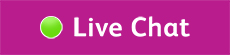# Based on my ticket type, how do you work out the compensation I’ll receive?

• #### Single and Return tickets

For a Single ticket, the cost of the ticket is the same as the cost of the journey. We work out the cost per journey of a Return ticket as half of the total ticket cost.

• #### Season tickets

If you have a Season ticket, we’ll work out the journey cost, as the ticket cost divided by the average number of working days. This will give us a daily rate of travel, which will then divide by two.

• #### Weekly tickets

We’ll divide the cost of the ticket by five working days to get the daily rate of travel. We’ll then divide this figure by two to get the cost of the journey – in simpler terms, your repayment will be the cost of the ticket divided by 10.

• #### Monthly tickets

We’ll divide the cost of the ticket by 20 working days. This will give us the daily rate of travel, before we divide this figure by two to achieve the cost of the journey. In simpler terms, the cost of your ticket is divided by 40.

• #### Annual tickets

We’ll divide the cost of the ticket by 232 working days (to get the daily rate of travel), before dividing it by two, to achieve the cost price of the journey. In simpler terms, the cost of the ticket will be divided by 464.

For Season tickets with other lengths of validity, we work out the number of working days (based on five in a full week) to achieve the journey cost, as above.

### Related Articles